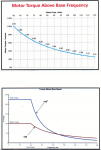# VFD- Input amperes are greater than Output

#### ghulamshoaib

Dear All,

Normally we see that on a VFD, input side amperes are less than output side amperes

but here in one case, input amps are more than output amps

can anyone tell me what may be the reason?

waiting

#### oneye14

You might say the input power is higher than the output power. If it's the other way round then we would ask what law of physics is involved..

Since electrical power is a function of voltage and currents, we can assume your input/output voltages are different; and since there is a large variety of VFD, both single phase and 3 phase, we would require a little more information to give a straight explanation.

#### PhilCorso

ghulamshoaib...
What type of ammeter are you using to determine amperes ?
Regards, Phil Corso

#### ghulamshoaib

You might say the input power is higher than the output power. If it's the other way round then we would ask what law of physics is involved..

Since electrical power is a function of voltage and currents, we can assume your input/output voltages are different; and since there is a large variety of VFD, both single phase and 3 phase, we would require a little more information to give a straight explanation.
Our VFD is 3 phase 380V
Amps on input side are higher than output

#### ghulamshoaib

ghulamshoaib...
What type of ammeter are you using to determine amperes ?
Regards, Phil Corso
Using normal clamp meter. And this meter is working fine with other drives

#### PhilCorso

ghulamshoaib...
So then, there is no question about it being a True Rms ammeter?
1) Have you measured all 3 phases?
2) What is Input to Output ampere Ratio ?
3) Do phase currents differ?
4) Can you measure Input to Output power with a clamp-on power meter?
5) What is Amperes in percent of rated capacity?
6) Is VFD "Auto-Tuning" type?

#### ghulamshoaib

ghulamshoaib...
So then, there is no question about it being a True Rms ammeter?
1) Have you measured all 3 phases?
2) What is Input to Output ampere Ratio ?
3) Do phase currents differ?
4) Can you measure Input to Output power with a clamp-on power meter?
5) What is Amperes in percent of rated capacity?
6) Is VFD "Auto-Tuning" type?
1. Yes, amps on all 3 phases are same
2. on 60Hz, input amps are 90A and output amps are 73A
3. No
4. I don't have power meter
5. output amps are of 75% of VFD rated capacity

waiting

#### joseph_e2

Frankly, I think I'd be more surprised to consistently see lower input current while operating at rated speed. At lower motor speeds, it's not unusual for a drive to also lower the voltage. If the output is 30 Hz and 240V, and the input is 480V, then I wouldn't be at all surprised to see that the output current is higher than input just because of the relationship between voltage, current, and power. At rated speed, I'd be surprised to see a lower input current since the output voltage should be very close to input and the drive is not 100% efficient.

#### InControl

Dear All,

Normally we see that on a VFD, input side amperes are less than output side amperes

but here in one case, input amps are more than output amps

can anyone tell me what may be the reason?

waiting
If the motor is running above base speed/frequency (extended frequency range), there might be some explanation. (Drawings borrowed from Delta Automation website)